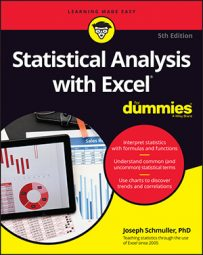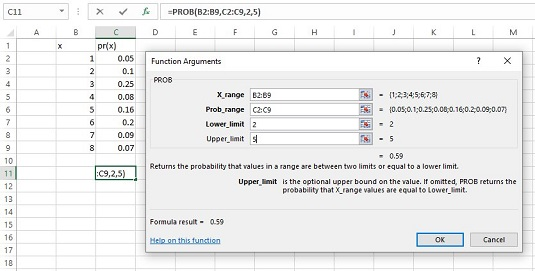##### Statistical Analysis with Excel For DummiesHere are some probability-related worksheet functions that you can use in Excel 2016 to help with statistical analysis. Although they’re a little on the esoteric side, you might find some use for them.

## PROB

If you have a probability distribution of a discrete random variable and you want to find the probability that the variable takes on a particular value, `PROB` is for you. Here is the `PROB` Argument Functions dialog box along with a distribution.The `PROB` Function Arguments dialog box and a probability distribution.

You supply the random variable (X_range), the probabilities (Prob_range), a lower limit, and an upper limit. `PROB` returns the probability that the random variable takes on a value between those limits (inclusive).

If you leave Upper Limit blank, `PROB` returns the probability of the value you gave for the lower limit. If you leave Lower Limit blank, `PROB` returns the probability of obtaining, at most, the upper limit (for example, the cumulative probability).

## WEIBULL.DIST

This is a probability density function that’s mostly applicable to engineering. It serves as a model for the time until a physical system fails. As engineers know, in some systems, the number of failures stays the same over time because shocks to the system cause failure. In others, like some microelectronic components, the number of failures decreases with time. In still others, wear-and-tear increases failures with time.

The Weibull distribution’s two parameters allow it to reflect all these possibilities. One parameter, Alpha, determines how wide or narrow the distribution is. The other, Beta, determines where it’s centered on the x-axis.

The Weibull probability density function is a rather complicated equation. Thanks to Excel, you don’t have to worry about it. The image below shows `WEIBULL.DIST`’s Function Arguments dialog box.

The dialog box answers the kind of question a product engineer would ask: Assume the time to failure of a bulb in an LCD projector follows a Weibull distribution with Alpha = .75 and Beta = 2,000 hours. What's the probability the bulb lasts at most 4,000 hours? The dialog box shows that the answer is .814.